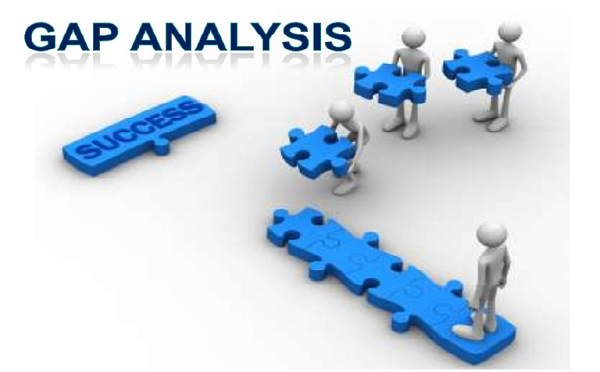Risk Measurement Techniques

In order to deal with the different types of risks involved in the management of assets and liabilities, we need to manage the risks for efficient bank management. There are various techniques used for measuring disclosure of banks to interest rate risks −

Gap Analysis Model

The gap analysis model portions the flow and level of asset liability mismatch through either funding or maturity gap. It is calculated for assets and liabilities of varying maturities and is derived for a set time horizon. This model checks on the repricing gap that is present in the middle of the interest revenue earned on the bank's assets and the interest paid on its liabilities within a mentioned interval of time.This model represents the total interest income disclosure of the bank, to variations occurring in the interest rates in different maturity buckets. Repricing gaps are estimated for assets and liabilities of varying maturities.

A positive gap reflects that assets are repriced before liabilities. Meanwhile, a negative gap reflects that liabilities need to be repriced before assets. The bank monitors the rate sensitivity that is the time the bank manager will have to wait so that there is a variation in the posted rates on any asset or liability of every asset and liability on the balance sheet.

The general formula that is used is as follows −

ΔNII = ΔRi×GAPi

In the above formula −

• NII is the total interest income.
• R is the interest rates influencing assets and liabilities in the relevant maturity bucket.
• GAP is the difference between the book value of the rate sensitive assets and the rate sensitive liabilities.

Hence, when there is a variation in the interest rate, we can easily analyze the influence of the variation on the total interest income of the bank. A change in interest rate has direct impact on their market value.

The main drawback of this model is that this method considers only the book value of assets and liabilities and thus neglects their market value. So, this method is an incomplete measure of the true interest rate exposure of a bank.

Duration Model

Duration or interval is a critical measure for the interest rate sensitivity of assets and liabilities. This is due to the fact that it considers the time of arrival of cash flows and the maturity of assets and liabilities. It is the measured average time to maturity of all the preset values of cash flows. This model states the average life of the asset or the liability. It is denoted by the following formula

DPp = D (dR /1+R)

The above equation briefs the percentage fall in price of the agreement for a given increase in the necessary interest rates or yields. The larger the value of the interval, the more sensitive is the cost of that asset or liability to variations in interest rates.

According to the above equation, the bank will be protected from interest rate risk if the duration gap between assets and the liabilities is zero. The major advantage of this model is that it uses the market value of assets and liabilities.

Simulation Model

This model assists in introducing a dynamic element in the examination of interest rate risk. The previous models — the Gap analysis and the duration analysis for asset-liability management endure from their inefficiency to move across the static analysis of current interest rate risk exposures. In short, the simulation models use computer power to support “what if” scenarios. For example,

What if

• the total level of interest rates switches
• marketing plans are under-achieved or over-achieved
• balance sheets shrink or expand

This develops the information available for management in terms of precise assessment of current exposures of asset and liability, portfolios to interest rate risk, variations in distributive target variables like the total interest income capital adequacy, and liquidity as well as the future gaps.

There are possibilities that this simulation model prevents the use to see all the complex paper work because of the nature of massive paper results. In this type of condition, it is very important to merge the technical expertise with proper awareness of issues in the enterprise.

There are particular demands for a simulation model to grow. These refer to accuracy of data and reliability of the assumptions or hypothesis made. In simple words, one should be in a status to look at substitutes referring to interest rates, growth-rate distributions, reinvestments, etc., under different interest rate scenes. This may be difficult and sometimes contentious.

An important point to note here is that the bank managers may not wish to document their assumptions and data is readily available for differential collision of interest rates on multiple variables. Thus, this model needs to be applied carefully, especially in the Indian banking system.

The application of simulation models addresses the commitment of substantial amount of time and resources. If in case, one can’t afford the cost or, more importantly the time engaged in simulation modeling, it makes perfect sense to stick to simpler types of analysis.

Useful Video Courses

Video

Learn Banking and Finance - Complete Course!

31 Lectures 3.5 hours